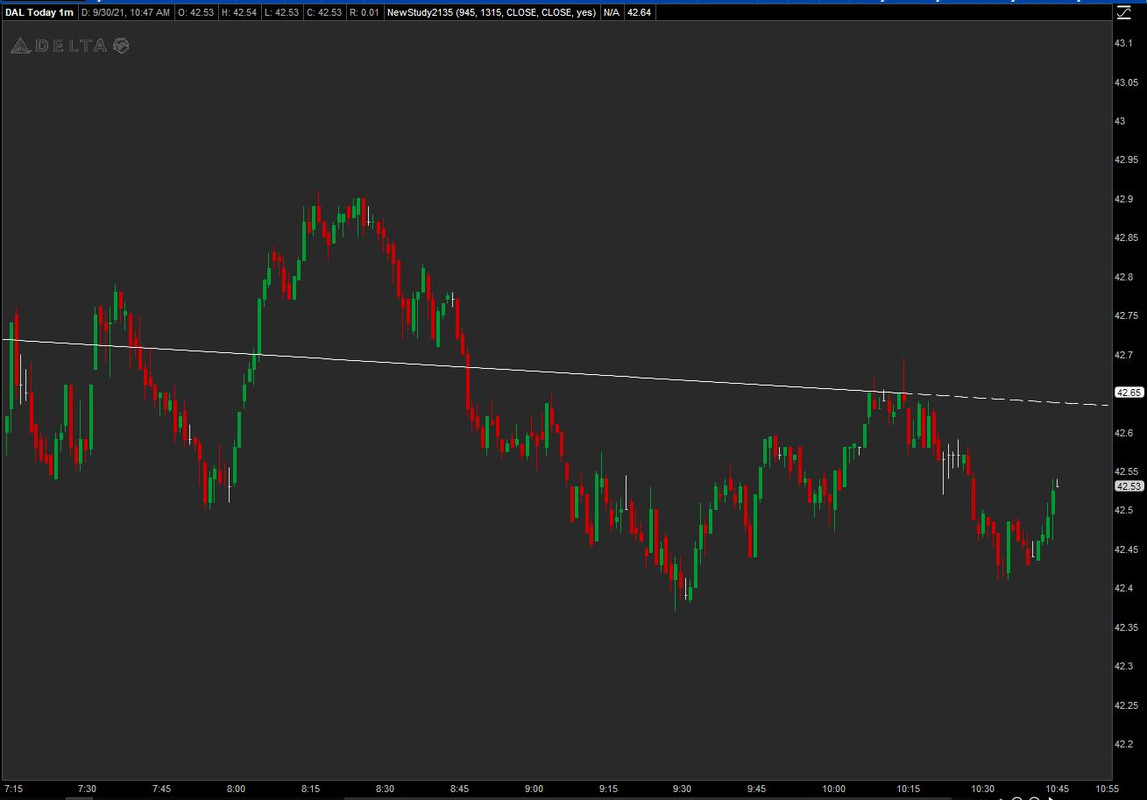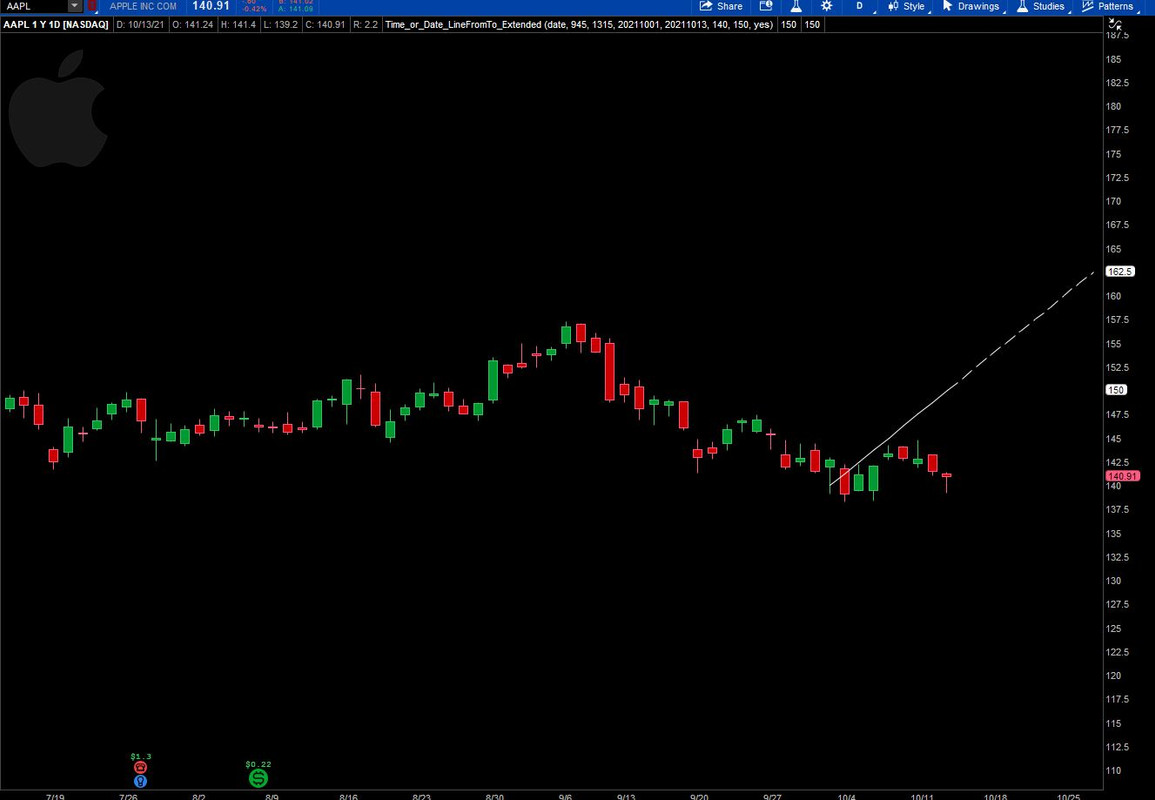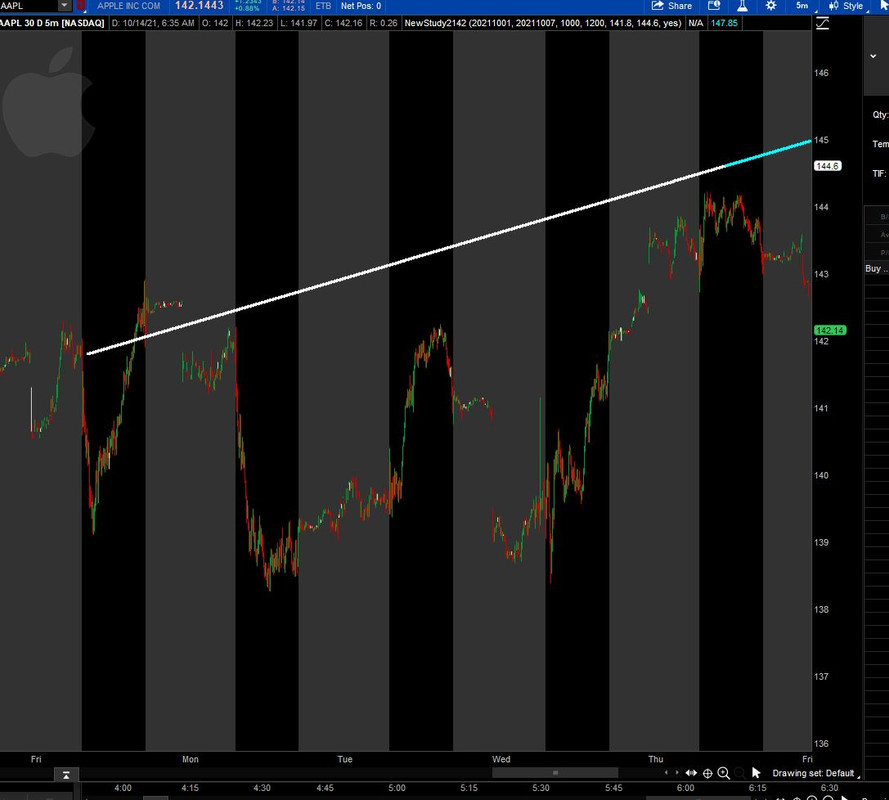# How To Plot A Line Between 2 Points

#### kaflesu

##### New member
Hi All,
Is there a way to plotline between 2 points in tos?

For example:

For stock "Citi" I want to draw a line between these points.
Begin point Value = 69.95 and date = "06/18/2021"
End point Value = 73 and date = "07/16/2021"

Solution
here is 1 way. you specify 2 dates and times and a price stat ( high, low, close, open) and it draws a lines between the 2 points

Ruby:
``````#https://jshingler.github.io/TOS-and-Thinkscript-Snippet-Collection/TOS%20&%20Thinkscript%20Collection.html#c-draw-a-line-between-two-price-points

#C-DRAW A LINE BETWEEN TWO PRICE POINTS

#Hint: Draws a line between to points defined by dates, times and price type
#Developed by RCG3 and StanL on the ThinkScript Lounge 1/2/14

input time1 = 1000; #hint time1:Time of first point using the 24-hour clock
input date1 = 20210302; #hint date1:Date of the first point in YYYYMMDD format
input time2 = 1300; #hint time2: Time of second point using the 24-hour clock
input date2 = 20210618; #hint date2: Date of the...``````

#### halcyonguy

##### Well-known member
VIP
here is 1 way. you specify 2 dates and times and a price stat ( high, low, close, open) and it draws a lines between the 2 points

Ruby:
``````#https://jshingler.github.io/TOS-and-Thinkscript-Snippet-Collection/TOS%20&%20Thinkscript%20Collection.html#c-draw-a-line-between-two-price-points

#C-DRAW A LINE BETWEEN TWO PRICE POINTS

#Hint: Draws a line between to points defined by dates, times and price type
#Developed by RCG3 and StanL on the ThinkScript Lounge 1/2/14

input time1 = 1000; #hint time1:Time of first point using the 24-hour clock
input date1 = 20210302; #hint date1:Date of the first point in YYYYMMDD format
input time2 = 1300; #hint time2: Time of second point using the 24-hour clock
input date2 = 20210618; #hint date2: Date of the first point in YYYYMMDD format
input price = high; #hint price: Select the price type desired

#====== To see dots on the inputted dates/times change the def below for

# ===== 'asdf' and 'asdf2' to plot and uncomment the setpaintingstrategy =====

def asdf= secondsfromtime(time1) == 0;
#asdf.setpaintingstrategy(paintingstrategy.boolean_points);
def asdf2= secondsfromtime(time2) == 0;
#asdf2.setpaintingstrategy(paintingstrategy.boolean_points);
def date= getyyyymmdd();

rec x1= if secondsfromtime(time1) == 0 and date1==date then barnumber() else x1;
rec x2= if secondsfromtime(time2) == 0 and date2==date then barnumber() else x2;
rec y1= if secondsfromtime(time1) == 0 and date1==date then price else y1;
rec y2= if secondsfromtime(time2) == 0 and date2==date then price else y2;
def x22= highestall(x2);
def y22= highestall(y2);
def slope= (y22-y1)/(x22-x1);

#plot line= if x1!=0 then y1+((barnumber()-x1)*slope) else double.nan;
plot line= if x1!=0 and x2==0 then y1+((barnumber()-x1)*slope) else double.nan;
def  Angle_deg = ATan(slope) * 180 / Double.Pi;

#addLabel(yes, "Slope = " +  Angle_deg + "degrees", color.CYAN);#Angle printout has no consistency at various aggs
#alert(price crosses line, "Price crossed trendline", alert.once,sound.chimes);# uncomment this line if used as a trendline``````

https://jshingler.github.io/TOS-and-Thinkscript-Snippet-Collection/TOS & Thinkscript Collection.html#c-draw-a-line-between-two-price-points
this site is an amazing resource with many explained examples
hal_2pts

Last edited:

#### TOS2221

##### New member
here is 1 way. you specify 2 dates and times and a price stat ( high, low, close, open) and it draws a lines between the 2 points

Ruby:
``````#https://jshingler.github.io/TOS-and-Thinkscript-Snippet-Collection/TOS%20&%20Thinkscript%20Collection.html#c-draw-a-line-between-two-price-points

#C-DRAW A LINE BETWEEN TWO PRICE POINTS

#Hint: Draws a line between to points defined by dates, times and price type
#Developed by RCG3 and StanL on the ThinkScript Lounge 1/2/14

input time1 = 1000; #hint time1:Time of first point using the 24-hour clock
input date1 = 20210302; #hint date1:Date of the first point in YYYYMMDD format
input time2 = 1300; #hint time2: Time of second point using the 24-hour clock
input date2 = 20210618; #hint date2: Date of the first point in YYYYMMDD format
input price = high; #hint price: Select the price type desired

#====== To see dots on the inputted dates/times change the def below for

# ===== 'asdf' and 'asdf2' to plot and uncomment the setpaintingstrategy =====

def asdf= secondsfromtime(time1) == 0;
#asdf.setpaintingstrategy(paintingstrategy.boolean_points);
def asdf2= secondsfromtime(time2) == 0;
#asdf2.setpaintingstrategy(paintingstrategy.boolean_points);
def date= getyyyymmdd();

rec x1= if secondsfromtime(time1) == 0 and date1==date then barnumber() else x1;
rec x2= if secondsfromtime(time2) == 0 and date2==date then barnumber() else x2;
rec y1= if secondsfromtime(time1) == 0 and date1==date then price else y1;
rec y2= if secondsfromtime(time2) == 0 and date2==date then price else y2;
def x22= highestall(x2);
def y22= highestall(y2);
def slope= (y22-y1)/(x22-x1);

#plot line= if x1!=0 then y1+((barnumber()-x1)*slope) else double.nan;
plot line= if x1!=0 and x2==0 then y1+((barnumber()-x1)*slope) else double.nan;
def  Angle_deg = ATan(slope) * 180 / Double.Pi;

#addLabel(yes, "Slope = " +  Angle_deg + "degrees", color.CYAN);#Angle printout has no consistency at various aggs
#alert(price crosses line, "Price crossed trendline", alert.once,sound.chimes);# uncomment this line if used as a trendline``````

https://jshingler.github.io/TOS-and-Thinkscript-Snippet-Collection/TOS & Thinkscript Collection.html#c-draw-a-line-between-two-price-points
this site is an amazing resource with many explained examples

Thank you for posting this.
How do I modify this to change the ending Y plot of the line to be a specified price value?
Ideally, I want the ending Y plot to be the value of a price level already plotted on the chart, but I'll be satisfied to get it from input price1.

#### Joshua

##### Well-known member
VIP
Ruby:
``````declare upper;
plot Line = if getYYYYMMDD() == 20210618 then 69.95
else if getYYYYMMDD() == 20210716 then 73
else double.nan;
Line.enableApproximation();``````

•d4dahl and halcyonguy

#### TOS2221

##### New member
Ruby:
``````declare upper;
plot Line = if getYYYYMMDD() == 20210618 then 69.95
else if getYYYYMMDD() == 20210716 then 73
else double.nan;
Line.enableApproximation();``````
I'm, not a programmer, but I don't understand why there's any if condition statement based on the date.
I'm looking for a script that draws a line from the close of the bar at a specified time to a time and price. For example, on a one-minute chart draw a line from the close at 9:45 to 4442.70 at 4:00.

#### SleepyZ

##### Well-known member
VIP
I'm, not a programmer, but I don't understand why there's any if condition statement based on the date.
I'm looking for a script that draws a line from the close of the bar at a specified time to a time and price. For example, on a one-minute chart draw a line from the close at 9:45 to 4442.70 at 4:00.
Josuha's script was just a great way to use what had been posted previously using dates. As you are now looking for intraday timing, see if this helps.

Ruby:
``````declare upper;
input priceto = 4442.70;
plot Line = if getday()==getlastday()
then
if secondsFromTime(0945)==0
then close
else if secondsfromTime(1600)==0
then priceto
else double.nan
else double.nan;
Line.enableApproximation();``````

#### Joshua

##### Well-known member
VIP
I'm, not a programmer, but I don't understand why there's any if condition statement based on the date.
I'm looking for a script that draws a line from the close of the bar at a specified time to a time and price. For example, on a one-minute chart draw a line from the close at 9:45 to 4442.70 at 4:00.

Well, I don't understand it either. If it is that specific, why not just draw it by hand with the line tool? The real question is; what conditions are being met at the start and end of the line that would make you even want to draw the line in the first place?

•#### MAHMOOD

##### New member
Hi,
Thanks for the code.

May I know how to expand the line to right? I mean once I draw a line between two points, how that can be automatically update as time progress?

Best regards

#### SleepyZ

##### Well-known member
VIP
Hi,
Thanks for the code.

May I know how to expand the line to right? I mean once I draw a line between two points, how that can be automatically update as time progress?

Best regards
Here is one way. Choose extendline and it will plot to the extension to the right edge of the chart.Ruby:
``````declare upper;

input from      = 0945;
input end       = 1315;
def linefrom    = SecondsFromTime(from) == 0;
def lineto      = SecondsFromTime(end) == 0;
input pricefrom = close;
input priceto   = close;
def  line       = if GetDay() == GetLastDay()
then if linefrom
then priceto
else if lineto
then pricefrom
else Double.NaN
else Double.NaN;
plot LL     = line;
LL.EnableApproximation();
LL.SetPaintingStrategy(PaintingStrategy.LINE);
LL.SetDefaultColor(Color.WHITE);

# Line Extended
input extendline = yes;

def cond1bn   = if linefrom then BarNumber() else cond1bn;
def cond2bn   = if lineto   then BarNumber() else cond2bn;

def y1        = if BarNumber() == HighestAll(cond1bn) then pricefrom else y1;
def y2        = if BarNumber() == HighestAll(cond2bn) then priceto   else y2;

def slope     = if cond2bn > cond1bn
then (( y2 - y1) / (cond2bn - cond1bn))
else (( y1 - y2) / (cond1bn - cond2bn));

def lext      = if cond2bn > cond1bn
then y1 + ((BarNumber() - cond1bn) * slope)
else y2 + ((BarNumber() - cond2bn) * slope);

plot lextplot = if extendline and BarNumber() >= HighestAll(cond2bn)
then lext else Double.NaN;
lextplot.SetStyle(Curve.MEDIUM_DASH);
lextplot.SetDefaultColor(Color.WHITE);
lextplot.SetLineWeight(1);``````

•halcyonguy and MAHMOOD

#### MAHMOOD

##### New member
Here is one way. Choose extendline and it will plot to the extension to the right edge of the chart

Here is one way. Choose extendline and it will plot to the extension to the right edge of the chart.
Thanks again. How can I assign with given two specific dates and prices (let say the first point is 10/01/2021 with price of \$100 and the second point is 10/13/2021 and the price is \$110) including the line extension.

Best regards

#### SleepyZ

##### Well-known member
VIP
Thanks again. How can I assign with given two specific dates and prices (let say the first point is 10/01/2021 with price of \$100 and the second point is 10/13/2021 and the price is \$110) including the line extension.

Best regards
Try this modification to the original script above to have a date option added to the time option to plot the line. The pricefrom and priceto can be values or price fundamentals.

I changed the values to fit better on an AAPL Daily chart for the dates you used.

[Ruby:
``````input method    = {default time, date};
input from      = 0945;
input end       = 1315;
input fromdate  = 20211001;
input todate    = 20211013;
input pricefrom = 100;
input priceto   = 110;

def linefrom    = SecondsFromTime(from) == 0;
def lineto      = SecondsFromTime(end)  == 0;
def datefrom    = getyyyyMMDD() == fromdate;
def dateto      = getyyyYMMDD() == todate;

def  linetime   = if GetDay() == GetLastDay()
then if linefrom
then priceto
else if lineto
then pricefrom
else Double.NaN
else Double.NaN;
def  linedate   = if  datefrom
then pricefrom
else if dateto
then priceto
else Double.NaN;
plot LL     = if method==method.time then linetime else linedate;
LL.EnableApproximation();
LL.SetPaintingStrategy(PaintingStrategy.LINE);
LL.SetDefaultColor(Color.WHITE);

# Line Extended
input extendline = yes;

def cond1bn   = if if method==method.time then linefrom else datefrom then BarNumber() else cond1bn;
def cond2bn   = if if method==method.time then lineto   else dateto   then BarNumber() else cond2bn;

def y1        = if BarNumber() == HighestAll(cond1bn) then pricefrom else y1;
def y2        = if BarNumber() == HighestAll(cond2bn) then priceto   else y2;

def slope     = if cond2bn > cond1bn
then (( y2 - y1) / (cond2bn - cond1bn))
else (( y1 - y2) / (cond1bn - cond2bn));

def lext      = if cond2bn > cond1bn
then y1 + ((BarNumber() - cond1bn) * slope)
else y2 + ((BarNumber() - cond2bn) * slope);

plot lextplot = if extendline and BarNumber() >= HighestAll(cond2bn)
then lext else Double.NaN;
lextplot.SetStyle(Curve.MEDIUM_DASH);
lextplot.SetDefaultColor(Color.WHITE);
lextplot.SetLineWeight(1);``````

#### MAHMOOD

##### New member
I copy and pasted the same code that you provided, and change the values for AAPL (141.8 and 144.6) but gave me a straight line all the way back to last year. I used daily timeframe as you had. Could not duplicate yours.

I am using also 5min time frame. Should it work for 5min time frame as well?

input method = {default time, date};
input from = 0945;
input end = 1315;
input fromdate = 20211001;
input todate = 20211007;
input pricefrom = 141.8;
input priceto = 144.6;

def linefrom = SecondsFromTime(from) == 0;
def lineto = SecondsFromTime(end) == 0;
def datefrom = getyyyyMMDD() == fromdate;
def dateto = getyyyYMMDD() == todate;

def linetime = if GetDay() == GetLastDay()
then if linefrom
then priceto
else if lineto
then pricefrom
else Double.NaN
else Double.NaN;
def linedate = if datefrom
then pricefrom
else if dateto
then priceto
else Double.NaN;
plot LL = if method==method.time then linetime else linedate;
LL.EnableApproximation();
LL.SetPaintingStrategy(PaintingStrategy.LINE);
LL.SetDefaultColor(Color.WHITE);

# Line Extended
input extendline = yes;

def cond1bn = if if method==method.time then linefrom else datefrom then BarNumber() else cond1bn;
def cond2bn = if if method==method.time then lineto else dateto then BarNumber() else cond2bn;

def y1 = if BarNumber() == HighestAll(cond1bn) then pricefrom else y1;
def y2 = if BarNumber() == HighestAll(cond2bn) then priceto else y2;

def slope = if cond2bn > cond1bn
then (( y2 - y1) / (cond2bn - cond1bn))
else (( y1 - y2) / (cond1bn - cond2bn));

def lext = if cond2bn > cond1bn
then y1 + ((BarNumber() - cond1bn) * slope)
else y2 + ((BarNumber() - cond2bn) * slope);

plot lextplot = if extendline and BarNumber() >= HighestAll(cond2bn)
then lext else Double.NaN;
lextplot.SetStyle(Curve.MEDIUM_DASH);
lextplot.SetDefaultColor(Color.WHITE);
lextplot.SetLineWeight(1);

#### SleepyZ

##### Well-known member
VIP
I copy and pasted the same code that you provided, and change the values for AAPL (141.8 and 144.6) but gave me a straight line all the way back to last year. I used daily timeframe as you had. Could not duplicate yours.
I have simplified the code so that it should work better on both intraday and daily charts. You will have to specify both date and time info to plot on intraday. Only should need dates for daily charts. The price to/from can be either price values or fundamentals.Ruby:
``````# Plots a line between two points to form a trendline, in this case two lows
# Added segment to extend/project the trend line to the right edge

input datefrom  = 20211001;
input dateto    = 20211007;
input timefrom  = 1000;
input timeto    = 1200;

input pricefrom = 141.8;#low;
input priceto   = 144.60;#low;

def linefrom = if GetYYYYMMDD() == datefrom and secondsfromTime(timefrom)==0 then 1 else 0;
def lineto   = if GetYYYYMMDD() == dateto   and secondsfromTime(timeto)==0   then 1 else 0;

plot line = if linefrom then pricefrom else if lineto then priceto else Double.NaN;
line.EnableApproximation();
line.SetDefaultColor(Color.WHITE);
line.SetLineWeight(3);

# Line Extended

def cond1bn = if linefrom then BarNumber() else cond1bn;
def cond2bn = if lineto   then BarNumber() else cond2bn;

rec y1 = if BarNumber() == HighestAll(cond1bn) then pricefrom else y1;
rec y2 = if BarNumber() == HighestAll(cond2bn) then priceto   else y2;

def slope = if cond2bn > cond1bn then (( y2 - y1) / (cond2bn - cond1bn)) else (( y1 - y2) / (cond1bn - cond2bn));

def lext  = if cond2bn > cond1bn then y1 + ((BarNumber() - cond1bn) * slope) else y2 + ((BarNumber() - cond2bn) * slope);

input showextendedline = yes;

plot lextplot = if showextendedline and BarNumber() >= HighestAll(cond2bn) then lext else Double.NaN;
lextplot.SetPaintingStrategy(PaintingStrategy.LINE);
lextplot.SetDefaultColor(Color.cyan);
lextplot.SetLineWeight(3);

# END STUDY``````

•MerryDay

#### MAHMOOD

##### New member
I have simplified the code so that it should work better on both intraday and daily charts. You will have to specify both date and time info to plot on intraday. Only should need dates for daily charts. The price to/from can be either price values or fundamentals.
Thanks

#### Williasmsmd19

##### New member
Hello, Is there a way to plot line with ext between, say... plot x and plot y## The Market Trading Game Changer

Join 2,500+ subscribers inside the useThinkScript VIP Membership Club
• Exclusive indicators
• Proven strategies & setups
• Private Discord community
• Exclusive members-only content
• 1 full year of unlimited support

What is useThinkScript?

useThinkScript is the #1 community of stock market investors using indicators and other tools to power their trading strategies. Traders of all skill levels use our forums to learn about scripting and indicators, help each other, and discover new ways to gain an edge in the markets.

How do I get started?

We get it. Our forum can be intimidating, if not overwhelming. With thousands of topics, tens of thousands of posts, our community has created an incredibly deep knowledge base for stock traders. No one can ever exhaust every resource provided on our site.

If you are new, or just looking for guidance, here are some helpful links to get you started.

What are the benefits of VIP Membership?